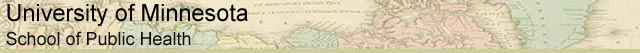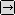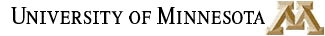### MATH REVIEW: USEFUL MATH FOR EVERYONE

SECTION 4. WHAT IS A LOGARITHM?

A logarithm is the power to which a number must be raised in order to get some other number (see Section 3 of this Math Review for more about exponents). For example, the base ten logarithm of 100 is 2, because ten raised to the power of two is 100:

log 100 = 2

because

102 = 100

This is an example of a base-ten logarithm. We call it a base ten logarithm because ten is the number that is raised to a power. The base unit is the number being raised to a power. There are logarithms using different base units. If you wanted, you could use two as a base unit. For instance, the base two logarithm of eight is three, because two raised to the power of three equals eight:

log2 8 = 3

because

23 = 8

In general, you write log followed by the base number as a subscript. The most common logarithms are base 10 logarithms and natural logarithms; they have special notations. A base ten log is written

log

and a base ten logarithmic equation is usually written in the form:

log a = r

A natural logarithm is written

ln

and a natural logarithmic equation is usually written in the form:

ln a = r

So, when you see log by itself, it means base ten log. When you see ln, it means natural logarithm (we'll define natural logarithms below). In this course only base ten and natural logarithms will be used.to Logarithms, Page 2